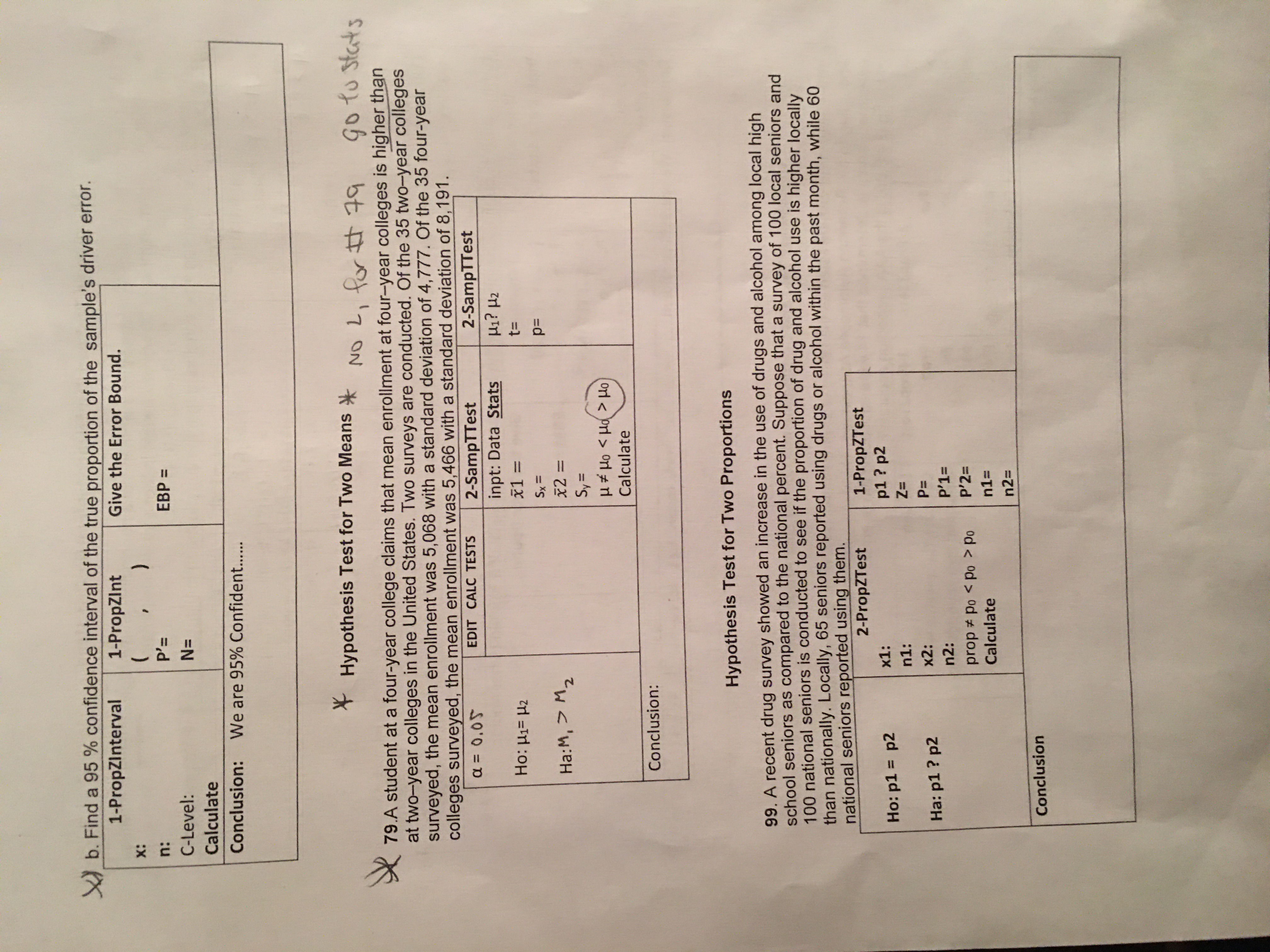# b. Find a 95 % confidence interval of the true proportion of the sample's driver error.1-PropZInterval1-PropZlntGive the Error Bound.( . )X:n:P'ЕВР 3C-Level:N=CalculateConclusion:We are 95% Confident.....Hypothesis Test for Two MeansNO L, fr t49Go to Stats79.A student at a four-year college claims that mean enrollment at four-year colleges is higher thanat two-year colleges in the United States. Two surveys are conducted. Of the 35 two-year collegessurveyed, the mean enrollment was 5,068 with a standard deviation of 4,777. Of the 35 four-yearcolleges surveyed, the mean enrollment was 5,466 with a standard deviation of 8,191.EDIT CALC TESTS2-SampTTestinpt: Data Stats2-SampTTesta 0.05Ho:H1 H2t=Sxp=Ha:M, M2x2=SyCalculateConclusion:Hypothesis Test for Two Proportions99. A recent drug survey showed an increase in the use of drugs and alcohol among local highschool seniors as compared to the national percent. Suppose that a survey of 100 local seniors and100 national seniors is conducted to see if the proportion of drug and alcohol use is higher locallythan nationally. Locally, 65 seniors reported using drugs or alcohol within the past month, while 60national seniors reported using them.1-PropZTestp1? p22-PropZTestх1:Ho: p1 = p2Z=n1:P=x2:Ha: p1? p2P'1=n2:P'2=prop Po < po > PoCalculaten1=n2=Conclusion

Question
39 views

Can you please answer question 79 and write the data from the calculator in the chart and write the conclusion thank youhelp_outlineImage Transcriptioncloseb. Find a 95 % confidence interval of the true proportion of the sample's driver error. 1-PropZInterval 1-PropZlnt Give the Error Bound. ( . ) X: n: P' ЕВР 3 C-Level: N= Calculate Conclusion: We are 95% Confident..... Hypothesis Test for Two Means NO L, fr t49 Go to Stats 79.A student at a four-year college claims that mean enrollment at four-year colleges is higher than at two-year colleges in the United States. Two surveys are conducted. Of the 35 two-year colleges surveyed, the mean enrollment was 5,068 with a standard deviation of 4,777. Of the 35 four-year colleges surveyed, the mean enrollment was 5,466 with a standard deviation of 8,191. EDIT CALC TESTS 2-SampTTest inpt: Data Stats 2-SampTTest a 0.05 Ho:H1 H2 t= Sx p= Ha:M, M2 x2= Sy Calculate Conclusion: Hypothesis Test for Two Proportions 99. A recent drug survey showed an increase in the use of drugs and alcohol among local high school seniors as compared to the national percent. Suppose that a survey of 100 local seniors and 100 national seniors is conducted to see if the proportion of drug and alcohol use is higher locally than nationally. Locally, 65 seniors reported using drugs or alcohol within the past month, while 60 national seniors reported using them. 1-PropZTest p1? p2 2-PropZTest х1: Ho: p1 = p2 Z= n1: P= x2: Ha: p1? p2 P'1= n2: P'2= prop Po < po > Po Calculate n1= n2= Conclusion fullscreen
check_circle

Step 1

Let four-year colleges be the first sample and the two-year colleges be the second sample.

Hypotheses for the test is given below:

Step 2

This test is a right-tailed test.

Test statistic for t-test:

Since population standard deviations are unknown, the appropriate test is two sample t-test.

Here, the sample means of four-year and two-year colleges are 5,466 and 5,068 respectively.

Sample standard deviations of four-year and two-year colleges are 8,191 and 4,777 respectively.

Sample size of both the samples are 35.

The pooled standard deviation can be calculated as follows:

Step 3

The test statistic for t-test is cal...

### Want to see the full answer?

See Solution

#### Want to see this answer and more?

Solutions are written by subject experts who are available 24/7. Questions are typically answered within 1 hour.*

See Solution
*Response times may vary by subject and question.
Tagged in

### Hypothesis Testing